Question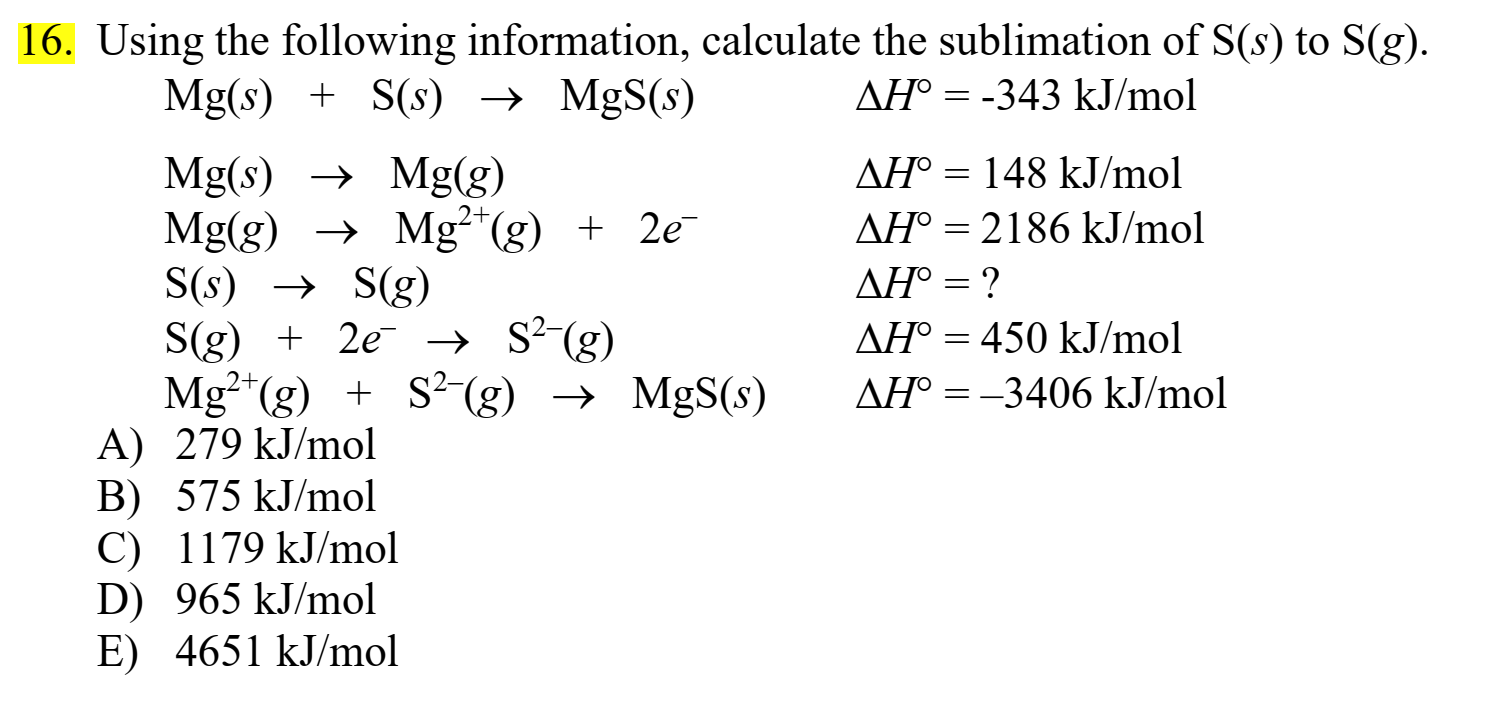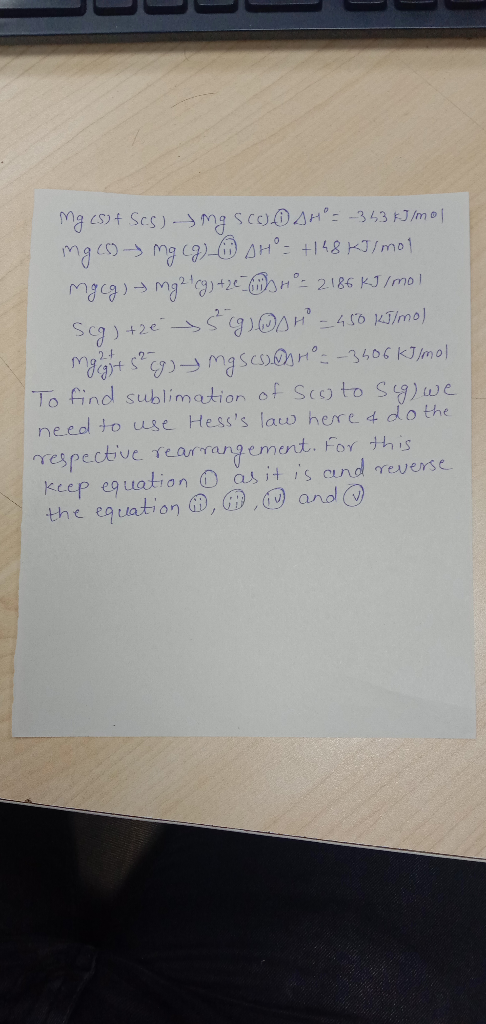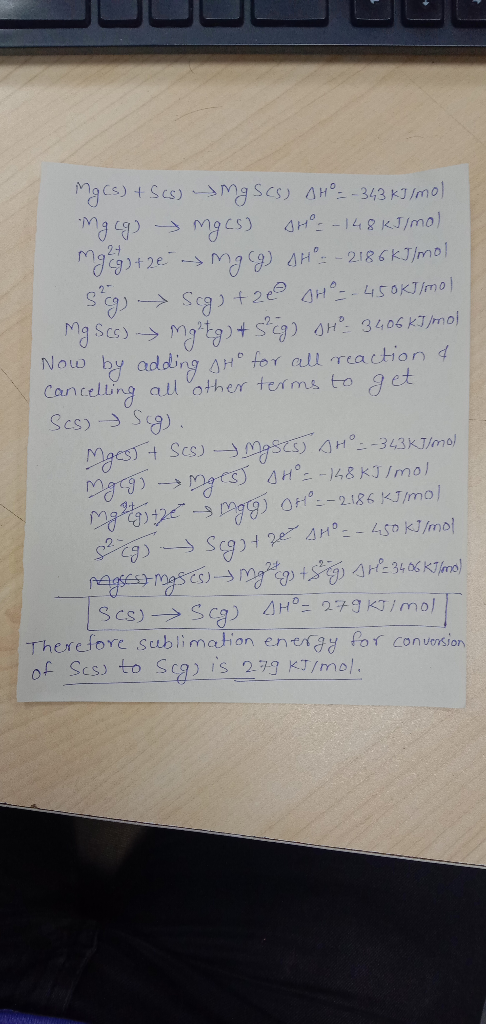If you find any mistake please mention in the comment box.

Thanks.

#### Earn Coins

Coins can be redeemed for fabulous gifts.

Similar Homework Help Questions
• ### 16. Using the following information, calculate the sublimation of S(s) to S(g). Mg(s) + S(s) + MgS(s) AH° = -343 kJ/mol...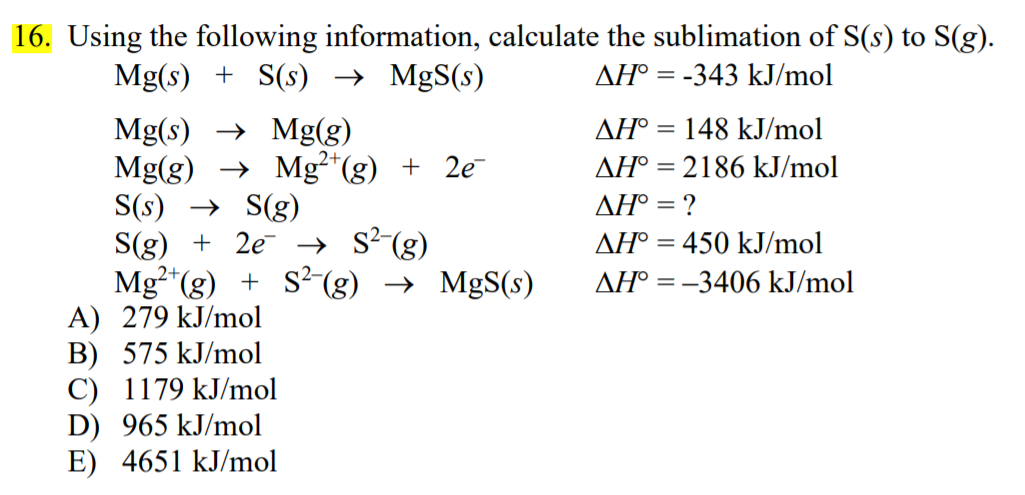16. Using the following information, calculate the sublimation of S(s) to S(g). Mg(s) + S(s) + MgS(s) AH° = -343 kJ/mol Mg(s) + Mg(g) AH° = 148 kJ/mol Mg(g) → Mg2+(g) + 2e AH° = 2186 kJ/mol S(s) + S(g) AH° = ? S(g) + 2e → S2-(g) AH° = 450 kJ/mol Mg2+(g) + SP-(g) + MgS(s) AH° = -3406 kJ/mol A) 279 kJ/mol B) 575 kJ/mol C) 1179 kJ/mol D) 965 kJ/mol E) 4651 kJ/mol

• ### Using the following information, calculate the heat of sublimation of S(s) to S(g). ?H°f MgS= -343...

Using the following information, calculate the heat of sublimation of S(s) to S(g). ?H°f MgS= -343 kJ/mol, ?H°sublimation Mg(s) = 148 kJ/mol, The lattice energy MgS = 3406 kJ/mol ?Mg(g) ? Mg2+(g) + 2 e ??st + 2nd IE = 2186 kJ/mol, S(g) +2e ? S2-(g) ????H = 450 kJ/mol, A. 575 kJ/mol B. 1179 kJ/mol C. 279 kJ/mol D. 965 kJ/mol E. 4651 kJ/mol

• ### 1. For each compound given here, write what type of compound it is (molecular or ionic...

1. For each compound given here, write what type of compound it is (molecular or ionic or acid). (a) NH4Cl (b) AlH3 (c) O2 (d) CH4 (e) NH3 (f) H2O (g) PCl5 (h) SF4 (I) ClF3 (j) XeF2 (k) SF6 (l) BrF5 (m) XeF4 (o) CS2 (p) CH2Cl2 2. Write a chemical equation that goes with Lattice energy of CaCl2(s). 3. Draw the Lewis Dot structure of .. (a) Na (b) Cl (c) O-2 (d) Mg+2 4. What is the...

• ### Consider the following data. Mg(s) + S(s) MgS(s) H = -598.0 kJ SO2(g) S(s) + O2(g)...

Consider the following data. Mg(s) + S(s) MgS(s) H = -598.0 kJ SO2(g) S(s) + O2(g) H = +296.8 kJ MgS(s) + 2 MgO(s) 3 Mg(s) + SO2(g) H = +1504.6 kJ Use Hess's law to calculate H for the reaction below. 2 Mg(s) + O2(g) 2 MgO(s)

• ### Calculate the standard enthalpy of formation of gaseous sulfur dioxide (S02) using the following thermochemical information:...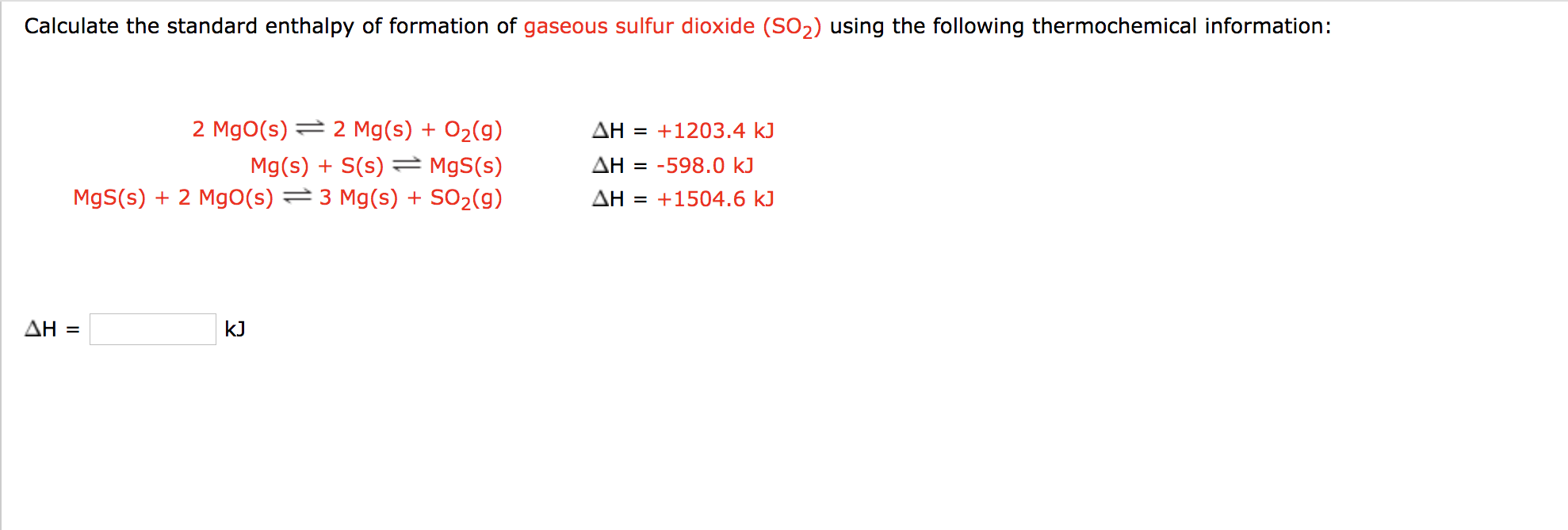Calculate the standard enthalpy of formation of gaseous sulfur dioxide (S02) using the following thermochemical information: 2 Mgo(s) = 2 Mg(s) + O2(g) Mg(s) + S(s) = MgS(s) Mgs(s) + 2 Mgo(s) = 3 Mg(s) + SO2(g) AH = +1203.4 k) AH = -598.0 kJ AH = +1504.6 kJ AH = k]

• ### 16. The sublimation of carbon dioxide at -78°C is given by: CO2(8) = CO2(g) AHsub =...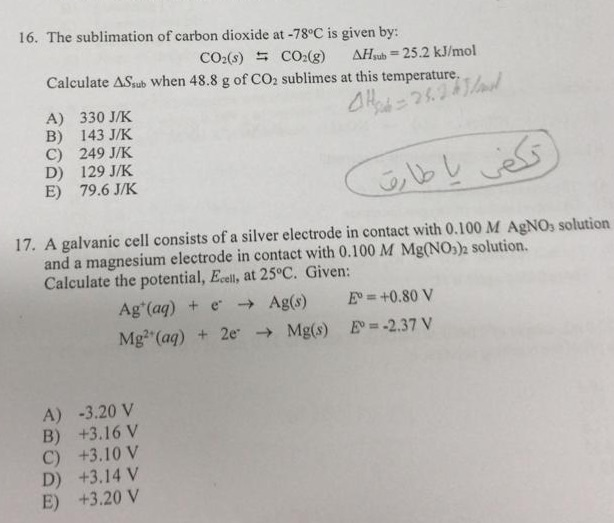16. The sublimation of carbon dioxide at -78°C is given by: CO2(8) = CO2(g) AHsub = 25.2 kJ/mol Calculate ASsub when 48.8 g of CO2 sublimes at this temperature AH-26.23 A) 330 J/K B) 143 J/K C) 249 J/K D) 129 J/K E) 79.6 JK 17. A galvanic cell consists of a silver electrode in contact with 0.100 M AgNO3 solution and a magnesium electrode in contact with 0.100 M Mg(NO3)2 solution. Calculate the potential, Ecell, at 25°C. Given: Ag...

• ### A chemist measures the enthalpy change AH during the following reaction: 2 HNO3(1) + Mg(s)—Mg(NO3),(s) +...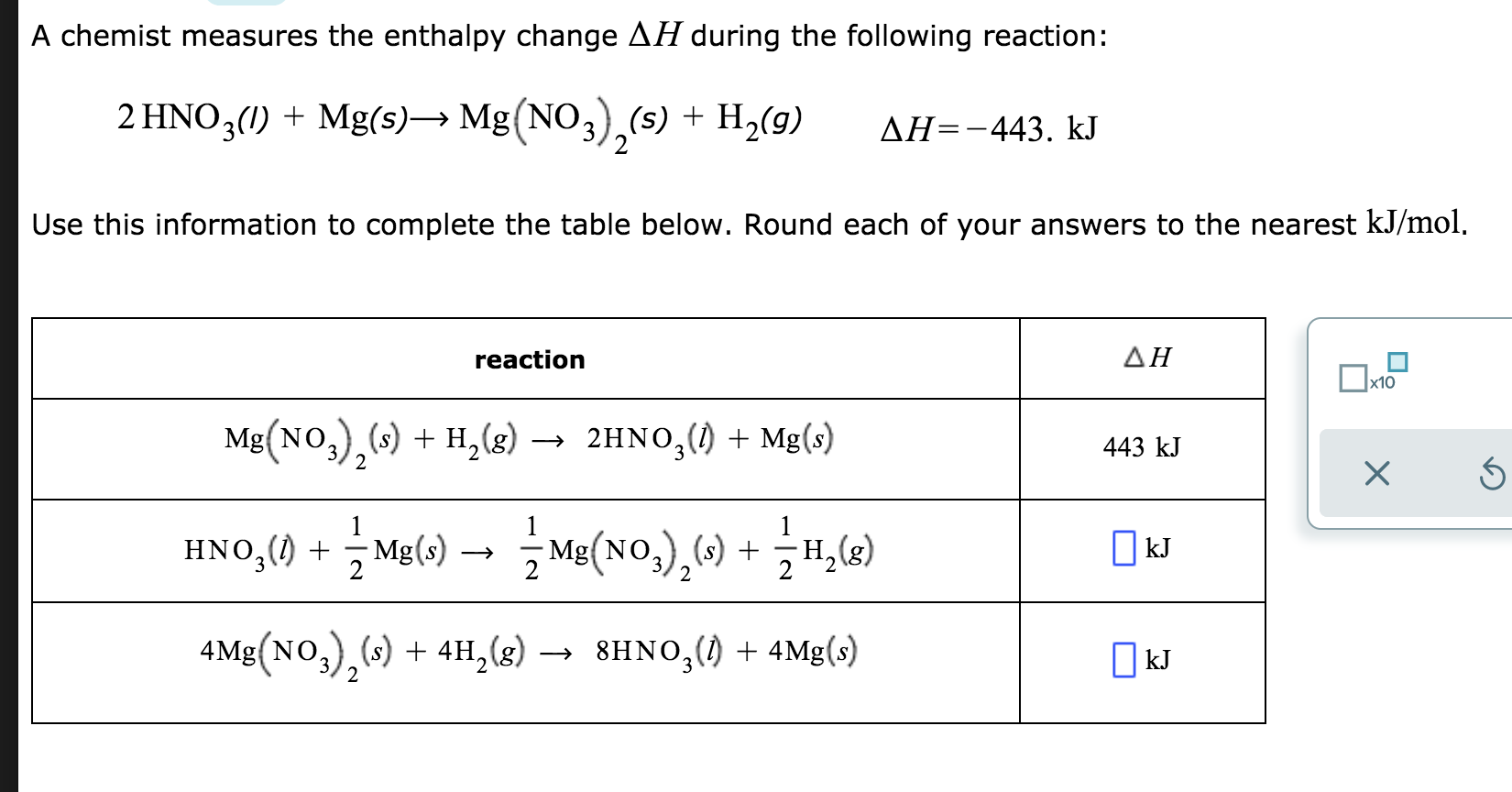A chemist measures the enthalpy change AH during the following reaction: 2 HNO3(1) + Mg(s)—Mg(NO3),(s) + H2(9) AH=-443. kJ Use this information to complete the table below. Round each of your answers to the nearest kJ/mol. reaction ΔΗ 443 kJ x 6 Mg(NO3),(s) + H2(g) → 2HNO3(1) + Mg(s) HN05(69) + Mg(s) – į Mg(NOx),() + <H() 4Mg(NO3),(s) + 4H, (8) — 8HN0g() + 4Mg(s) [kJ OkJ

• ### Pre-lab Questions for Experiment #11 Hess's Law with Computers 1) Using the following data, NaOH ()...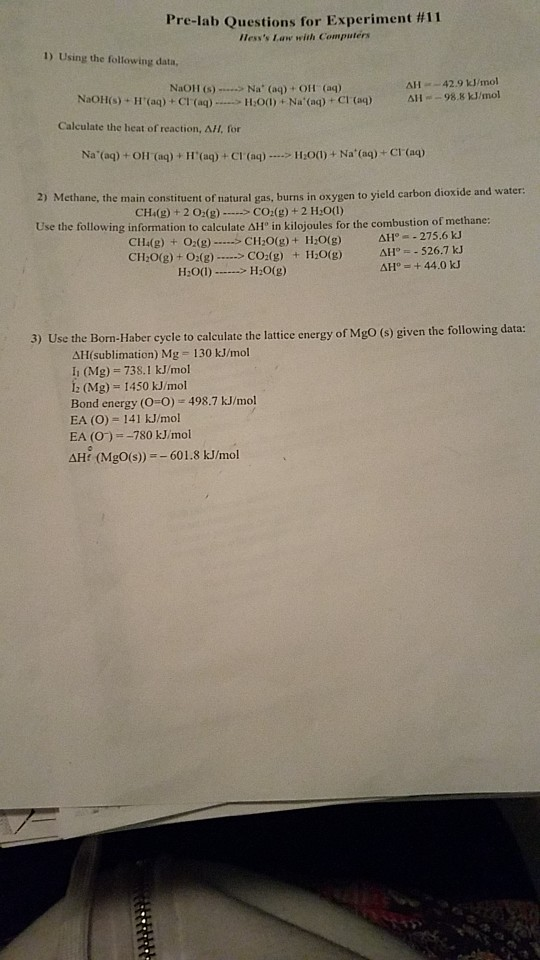Pre-lab Questions for Experiment #11 Hess's Law with Computers 1) Using the following data, NaOH () - Na' (aq) + OH (aq) ΔΙΙ "-42.9 kJ/mol =-98.8 kJ/mol NaOHis).HUaq) + Cl.(aq)....-11:0(1) + Na. (ap-Craq) Calculate the heat of reaction, AH, for ΔΙΙ 2) Methane, the main constituent of natural gas, burns in oxygen to yield carbon dioxide and water: CH(g) + 2 02(g)CO(g) +2 H:00) Use the following information to calculate ΔΗ, in kilojoules for the combustion of methane CH(g) +...

• ### A chemist measures the enthalpy change AH during the following reaction: 2 HNO3(1) + Mg(s) Mg(NO3),(s)...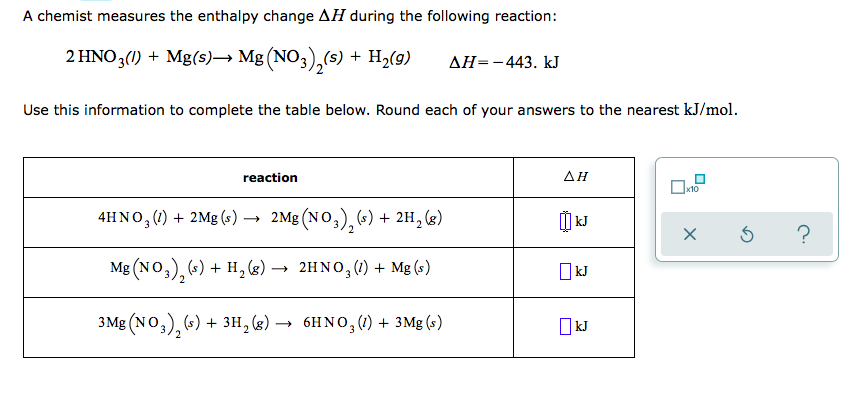A chemist measures the enthalpy change AH during the following reaction: 2 HNO3(1) + Mg(s) Mg(NO3),(s) + H2(9) AH=-443. kJ Use this information to complete the table below. Round each of your answers to the nearest kJ/mol. reaction ΔΗ 4HN03(1) + 2Mg () — 2Mg(NO3),(5) + 2H, (6) Mg(NO3), (5) + H, (8) — 24NO3 (1) + Mg () xs ? kJ 3Mg(NO3), (s) + 3H, (8) — 6HNO3(1) + 3Mg(s)

• ### Using the Born-Haber cycle shown below, calculate the lattice energy for MgCl2 in kJ* mol-1 Mg**g)...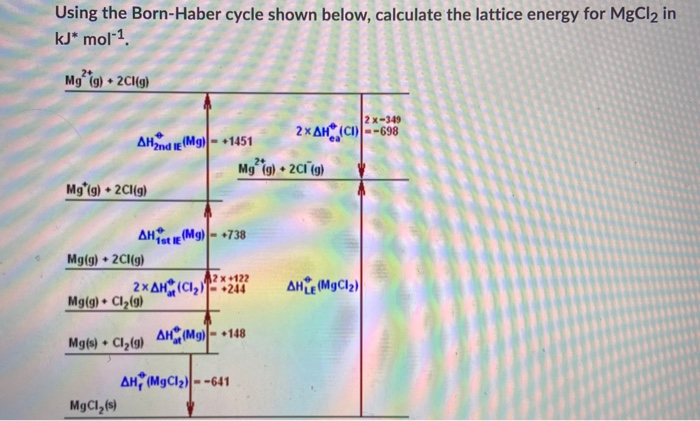Using the Born-Haber cycle shown below, calculate the lattice energy for MgCl2 in kJ* mol-1 Mg**g) + 2Cl(g) 2 x-349 AH2nd le(Mg) - 1451 2xAH (01) --698 My*(g) 2013) Mg (g) + 2Cl(g) AHORE (Mg) - 738 Mg(g) + 2Cl(g) 2 x 122 2xAH CIT- +244 Mg(g) + Cl2(g) AHTE (MgCl2) Mg(s) + Clą(9) AHM9) - 148 AH, (MgCl2) --641 MgCl (s)